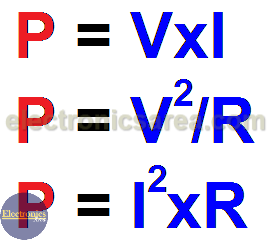# Energy and Electric power

Electrical energy is the energy resulting from the flow of electric charge.  Electrical energy is usually encountered as potential energy, which is energy stored due to the relative positions of charged particles or electric fields.

Electric power is the rate, per unit of time, at which electrical energy is transferred by an electric circuit or the Electric power is the rate or speed at which work is done. Electric power is the multiplication of the electric current (amps) by the voltage (volts). P = I x V (watts). Using the Ohm’s law (V = IxR) we obtain P = [V/R]xV. P = V2/R  and P = [I/R] x I. P = I2xR .

For example: What is the power dissipated in a resistor if the current I is 0.25 amperes and the voltage V is 3 volts.

The power is: P = I x V = 0.25 x 3 = 0.75 watts = 750 milliwatts.

Using the #2 formula we can get electric power without the current value and, using the #3 formula the power is obtained without the voltage value. These formulas are useful for finding the power in a resistor, where the energy is converted into heat.But not always electricity is converted into heat. In an electric motor, the power is converted mainly into mechanical motion. In radio and television stations, power largely become, electromagnetic waves. In a stereo, power becomes sound waves. In a bulb power becomes light and heat.

Normally the power converted into heat, is considered lost power or useless power. The main idea is that the power supplied is make the most, and that the power lost in heat and others is minimal. To find out how well the power is used, we use the term “Performance”.

## What is Performance?

Performance = Output power / Input power.

For example: What the performance is if we use a 100 watts (input power) light bulb, and the power transformed into light is 80 watts (output power), performance is: 80/100 = 0.8 = 80%. The remaining 20% is lost in heat.

The unit of electrical power is watt. Multiples and submultiples of watt are:

• 1 nanowatt = 1 nW = 1 watt / 1 000 000 000.
• 1 microwatt = 1 uW = 1 watt / 1000 000.
• 1 milliwatt = 1 mW = 1 watt / 1000.
• 1 kilowatt = 1 Kw = 1000 watts.
• 1 megawatt = 1 MW = 1000 kilowatts = 1 000 000 watts.
• 1 gigawatt = 1 GW = 1 000 MW = 1 000 000 000 watts.
• 1 Terawatt = 1 TW = 1 000 000 MW = 1 000 000 000 000 watts.

Note: 1 HP (Horsepower) = 745.7 watts.

• 2
•
•
•
•
•
•
•
2
Shares
•
2
Shares
• 2
•
•
•
•
•
•